Courses

# RD Sharma Solutions -Ex-6.8, Fractions, Class 6, Maths Class 6 Notes | EduRev

## RD Sharma Solutions for Class 6 Mathematics

Created by: Abhishek Kapoor

## Class 6 : RD Sharma Solutions -Ex-6.8, Fractions, Class 6, Maths Class 6 Notes | EduRev

The document RD Sharma Solutions -Ex-6.8, Fractions, Class 6, Maths Class 6 Notes | EduRev is a part of the Class 6 Course RD Sharma Solutions for Class 6 Mathematics.
All you need of Class 6 at this link: Class 6

Q. 1. Write these fractions appropriately as additions or subtraction :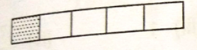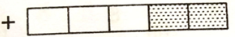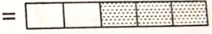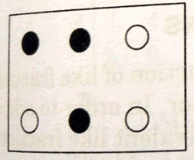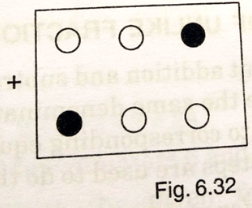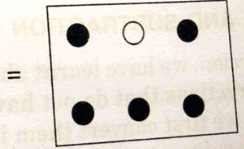Q. 2. Solve : i)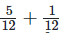ii)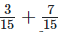iii)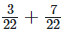iv)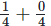v)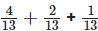vi)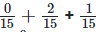vii)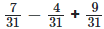viii)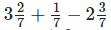ix)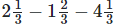x)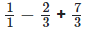xi)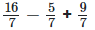Sol : i) The given fractions are: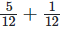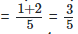Hence the answer is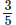ii) The given fractions are: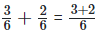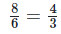Hence the answer is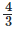iii) the given fractions are :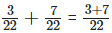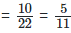Hence the answer is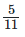iv) the given fractions are :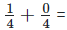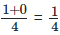Hence the answer is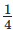v) The given fractions are :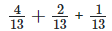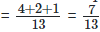Hence the answer is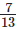vi) the given fractions are :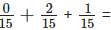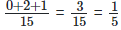Hence the answer is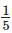vii) the given fractions are :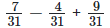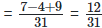Hence the answer is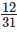viii) the given fractions are :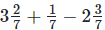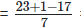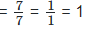Hence the answer is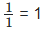ix) the given fractions are :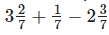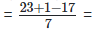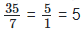Hence the answer is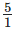= 5
x) the given fractions are :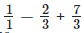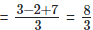Hence the answer is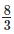xi ) the given fractions are :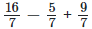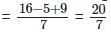Hence the answer is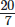Q 3. Shikha painted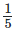of the wall space in her room. Her brother ravish helped and painted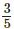of the wall space. How much did they paint together? How much the room is left unpainted?
Sol : Shikha paintedof the wall space in her room Ravish painted 35 of the wall space Wall space painted by both of them together =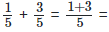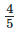Unpainted part of the room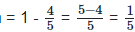Q 4. Ramesh bought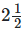kg sugar whereas rohit bought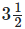kg of sugar. Find the total amount of sugar bought by both of them.
Sol : Quantity of sugar bought by ramesh =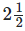kg =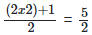kg
Quantity of sugar bought by rohit =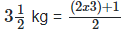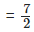kg
Total amount of sugar bought by them : Quantity of sugar bought by rohit + Quantity of sugar bought by ramesh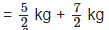= 6 kg ( Dividing numerator and denominator by their HCF ( 6 ) )

Q 5. The teacher taught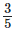of the book, Vivek revisedmore on his own. How much does he still have to revise ?
Sol : Fraction of the book taught by the teacher =Fraction of the book revised by vivek =Fraction of the book still left for revision by vivek :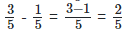Therefore, Fraction of the book still left for revision by vivek is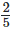Q 6. Amit was given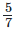of a bucket of oranges. What fraction of oranges was left in the basket ?
Sol : Fraction of oranges given to amit =Fraction of oranges left in the basket :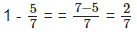Therefore, Fraction of oranges left in the basket is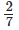Q 7. Fill in the missing fractions : i)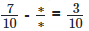ii)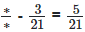iii)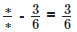iv)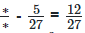sol : i) Given :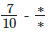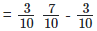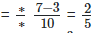Therefore,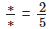ii) Given :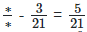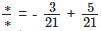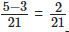Therefore,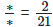iii) Given :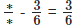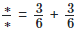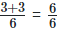Therefore,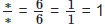iv) Given :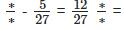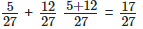Therefore,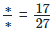103 docs

,

,

,

,

,

,

,

,

,

,

,

,

,

,

,

,

,

,

,

,

,

,

,

,

,

,

,

,

,

,

;